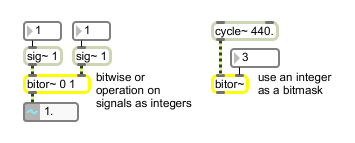# bitor~

Bitwise or-operation of floating point signals

## Description

The bitor~ object performs a bitwise "or" on two incoming floating-point signals as either raw 32-bit data or as integer values. The bits of both incoming signals are compared, and a 1 is output if either of the two bit values is 1. The output is a floating-point signal composed of the resulting bit pattern.

## Arguments

Name Type Opt Description
bitmask int opt Sets the bitmask to be used by the bitor~ object. The default is 0. An integer value can be used as a bitmask regardless of the mode; the binary representation of this integer is the bitmask.
operational-mode (0 through 3) int opt Specifies whether the floating-point signal or floating-point values will be processed as raw 32-bit floating-point values or converted to integer values for the bitwise operation. The modes of operation are:
0: Treat both floating-point signal inputs as raw 32-bit values (default).
1: Convert both floating-point signal inputs to integer values.
2: Treat the floating-point signal in the left inlet as a raw 32-bit value and treat the value in the right inlet as an integer.
3: Convert the floating-point signal in the left inlet to an integer and treat the right input as a raw 32-bit value.

Note: If you convert the floating-point signal input to an integer and then convert it back, the resulting floating-point value will retain only 24 bits of integer resolution.

## Messages

 int bitmask [int] In right inlet: An integer value can be used as a bitmask when supplied to the right inlet of the bitor~ object, provided that the proper mode is set. float bitmask [float] Performs the same function as int. bits bitmask (32 ones or zeros) [list] In left inlet: The word bits, followed by a list containing 32 ones or zeros, specifies a bitmask to be used by bitor~. Alternately, a bitmask value can be set by using an int value in the right inlet. mode operational-mode (0 through 3) [int] In left inlet: The word mode, followed by a zero or one, specifies whether the floating signal or floating-point values will be processed as a raw 32-bit floating-point value or converted to an integer value for the bitwise operation. The modes of operation are: Mode Descriptions: 0 - Treat both floating-point signal inputs as raw 32-bit values (default). 1 - Convert both floating-point signal inputs to integer values. 2 - Treat the floating-point signal in the left inlet as a raw 32-bit value and treat the value in the right inlet as an integer. 3 - Convert the floating-point signal in the left inlet to an integer and treat the right input as a raw 32-bit value. Note: If you convert the floating-point signal input to an integer and then convert it back, the resulting floating-point value will retain only 24 bits of integer resolution. signal In left inlet: The floating-point signal is compared, in binary form, with the floating-point signal in the right inlet. The signal can be treated as either a floating-point signal or as an integer. In right inlet: The floating-point signal to be compared with the signal in the left inlet. The signal can be treated as either a floating-point signal or as an integer. The raw floating-point signal bit values are expressed in the following form: <1 sign bit> <8 exponent bits> <23 mantissa bits>

## Output

signal: The two floating-point signals or ints received in the inlets are compared, one bit at a time. If a bit is 1 in either one of the numbers, it will be 1 in the output number, otherwise it will be 0 in the output number. The output is a floating-point signal composed of the resulting bit pattern.

## Examples